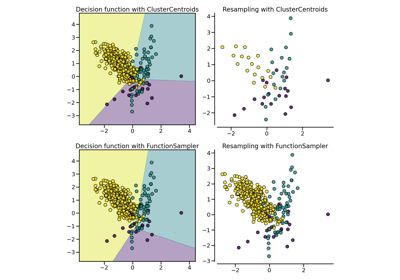# `imblearn.under_sampling`.OneSidedSelection¶

class `imblearn.under_sampling.``OneSidedSelection`(*, sampling_strategy='auto', random_state=None, n_neighbors=None, n_seeds_S=1, n_jobs=None)[source]

Class to perform under-sampling based on one-sided selection method.

Read more in the User Guide.

Parameters
sampling_strategystr, list or callable

Sampling information to sample the data set.

• When `str`, specify the class targeted by the resampling. Note the the number of samples will not be equal in each. Possible choices are:

`'majority'`: resample only the majority class;

`'not minority'`: resample all classes but the minority class;

`'not majority'`: resample all classes but the majority class;

`'all'`: resample all classes;

`'auto'`: equivalent to `'not minority'`.

• When `list`, the list contains the classes targeted by the resampling.

• When callable, function taking `y` and returns a `dict`. The keys correspond to the targeted classes. The values correspond to the desired number of samples for each class.

random_stateint, RandomState instance, default=None

Control the randomization of the algorithm.

• If int, `random_state` is the seed used by the random number generator;

• If `RandomState` instance, random_state is the random number generator;

• If `None`, the random number generator is the `RandomState` instance used by `np.random`.

n_neighborsint or object, default=None

If `int`, size of the neighbourhood to consider to compute the nearest neighbors. If object, an estimator that inherits from `sklearn.neighbors.base.KNeighborsMixin` that will be used to find the nearest-neighbors.

n_seeds_Sint, default=1

Number of samples to extract in order to build the set S.

n_jobsint, default=None

Number of CPU cores used during the cross-validation loop. `None` means 1 unless in a `joblib.parallel_backend` context. `-1` means using all processors. See Glossary for more details.

`EditedNearestNeighbours`

Undersample by editing noisy samples.

Notes

The method is based on .

Supports multi-class resampling. A one-vs.-one scheme is used when sampling a class as proposed in . For each class to be sampled, all samples of this class and the minority class are used during the sampling procedure.

References

1(1,2)

M. Kubat, S. Matwin, “Addressing the curse of imbalanced training sets: one-sided selection,” In ICML, vol. 97, pp. 179-186, 1997.

Examples

```>>> from collections import Counter
>>> from sklearn.datasets import make_classification
>>> from imblearn.under_sampling import     OneSidedSelection
>>> X, y = make_classification(n_classes=2, class_sep=2,
... weights=[0.1, 0.9], n_informative=3, n_redundant=1, flip_y=0,
... n_features=20, n_clusters_per_class=1, n_samples=1000, random_state=10)
>>> print('Original dataset shape %s' % Counter(y))
Original dataset shape Counter({1: 900, 0: 100})
>>> oss = OneSidedSelection(random_state=42)
>>> X_res, y_res = oss.fit_resample(X, y)
>>> print('Resampled dataset shape %s' % Counter(y_res))
Resampled dataset shape Counter({1: 496, 0: 100})
```
Attributes
sample_indices_ndarray of shape (n_new_samples)

Indices of the samples selected.

New in version 0.4.

`__init__`(self, *, sampling_strategy='auto', random_state=None, n_neighbors=None, n_seeds_S=1, n_jobs=None)[source]

Initialize self. See help(type(self)) for accurate signature.

## Examples using `imblearn.under_sampling.OneSidedSelection`¶